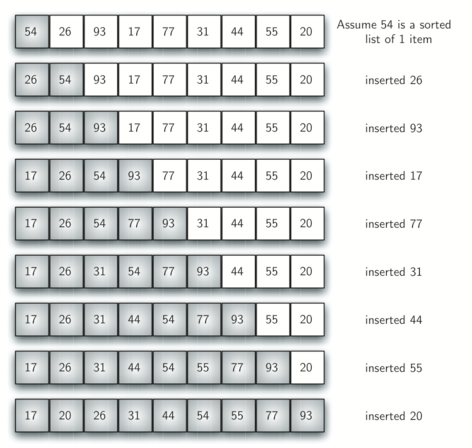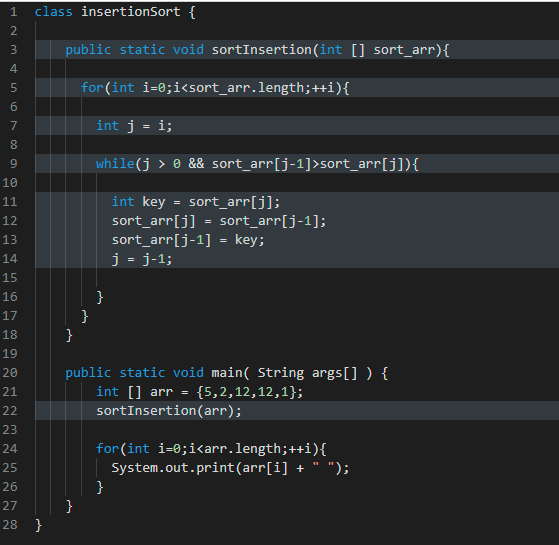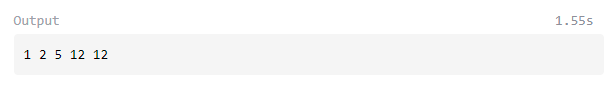HomeBlogSoftware DevelopmentInsertion Sort in Java: Explained with Examples

# Insertion Sort in Java: Explained with Examples

Last updated:
31st May, 2023
Views
7 MinsView All### Basics of Sorting Algorithms in Java

Sorting refers to the arrangement of elements in a specific order, often used in data structures such as arrays. The criteria in which the elements are sequenced is diverse. The most common ones are the sorting of numbers in ascending and descending order, lexicographical sorting of strings etc. Some of the most common and effective sorting algorithms used in Java and other object-oriented programming languages are:

• Bubble sort
• Selection sort
• Insertion sort
• Merge sort
• Quicksort
• Heapsort

Insertion sort is an easy-to-understand sorting algorithm whose working is similar to the manner we play cards. The input array list is split into two parts: sorted and unsorted. The splitting is virtual. The items from the unsorted part of the list are shifted to the sorted portion one at a time in each iteration.

Learn Software Development Courses online from the World’s top Universities. Earn Executive PG Programs, Advanced Certificate Programs or Masters Programs to fast-track your career.

## Implementation of Insertion Sort in Java

In insertion sort, the current element is compared with the immediate next element in each pass. The comparison is made to determine if the element is greater than the element it is compared to. If the item is greater than the one it is compared to, it is retained in the same position and the iteration continues. If the item is lesser than the one it is compared to, it is shifted to its appropriate position by shifting the larger items by one position backwards.

## An example for Insertion Sort## Figure 1 Insertion Sort Example

In the insertion sorting algorithm, the first iteration starts with an assumption that the item at the 0th position is already sorted. For each iteration, the current item is checked against the items in the sorted region of the list. The items that are greater during the comparison are moved towards the right of the list. In this algorithm, the current item can be inserted when the smaller item is reached.

## Explore our Popular Software Engineering Courses

 Master of Science in Computer Science from LJMU & IIITB Caltech CTME Cybersecurity Certificate Program Full Stack Development Bootcamp PG Program in Blockchain Executive PG Program in Full Stack Development View All our Courses Below Software Engineering Courses

Figure 1 shows an example of an insertion sort with a list of items. In the first iteration, the element  is assumed to be sorted already. This acts as a reference for the comparison during the first iteration. The second element  is less than 54 and hence, 54 is moved to the right.

Check out our free technology courses to get an edge over the competition.

## Example Java Code for an Insertion Sort

Now that we have gained knowledge about the working of the insertion sort algorithm let’s visualize how a Java code is written to sort the elements with this algorithm. In the example code shown below, the values of arr can be changed as per your needs and how the program responds for different input arrays.The output of the above code is mentioned below.Explanation of the implemented Java code:

• As an initial step in the program, a method to accept the input array to be sorted is created. It is referred to as sort_arr in the above code.
• Secondly, an outer for loop is created to iterate over each element of the input array, as reflected in line 5 of the above code.
• The next step is the creation of a distinct iterator ‘j’ to evaluate for the sorting of the elements in ascending order of the input list. This is shown in the 7th line of the code.
• In the next step, an inner while loop is created.
• The while loop is expected to meet two specified conditions as in line 9 of the code. The two conditions are that the j’s value must be more than 0 and the j-1 index value must be more than the j’s index value.
• If both the conditions evaluate to true, lines 11 to 14 are executed and the j’s index value is assigned as the key’s value.
• The values at j-1 and j are exchanged.
• j’s value decrements by 1 and the loop repeats till any of the two conditions is evaluated to be false.
• The execution continues for each traverse of the outer for loop till the condition for that also breaks.

## In-Demand Software Development Skills

 JavaScript Courses Core Java Courses Data Structures Courses Node.js Courses SQL Courses Full stack development Courses NFT Courses DevOps Courses Big Data Courses React.js Courses Cyber Security Courses Cloud Computing Courses Database Design Courses Python Courses Cryptocurrency Courses

## Advantages of Insertion sort in Java

• Insertion sort in Java is a purely simple sorting algorithm.
• There is no change in the comparative order of constituents of the list.
• This sorting algorithm is able to sort the list as and when the list is received.
• This sorting algorithm works effectively for small datasets.
• The algorithm demands a constant quantity of extra space or memory.

## Read our Popular Articles related to Software Development

 Why Learn to Code? How Learn to Code? How to Install Specific Version of NPM Package? Types of Inheritance in C++ What Should You Know?

## Insertion Sort In Java

Insertion sort is an efficient sorting algorithm that allows for in-place sorting of an array.

It follows one element at a time. In this sorting approach, the array is changed without any temporary structures.

### Characteristics Of Insertion Sort In Java

• The implementation of this method is straightforward, making it one of the simplest.
• In general, insert sort is effective for small data values.
• The nature of insertion sort is adaptive.
• Because linked lists contain pointers to both the next (singly linked list) and previous (doubly linked list) elements, insertion sort operations on them are simpler.
• The insertion sort algorithm Java is frequently used in the following situations:
• The array only has a few elements.
• Only a few elements remain to sort.

### Pseudocode

Below is the pseudocode for insertion sort in Java

```INSERTION-SORT(array)

for i=2 to array.length

key_element = array[i]

j = i - 1

while j > 0 and array[j] > key_element

array[j+1] = array[j]

j = j - 1

array[j + 1] = key_element

```

Let’s quickly review the algorithm mentioned above.

• The current item in the array to be processed is indicated by the index i.
• As an array with only one item is, by definition, considered to be sorted, we start with that item.
• A key is the thing that is at index i.
• The second step of the technique involves determining the right index once the key has been obtained.
• The key shifts one position to the left if it exceeds the item’s value at index j. Up until the point where an element is smaller than the key, the operation continues.

### Recursive approach

In the recursive approach of Insertion Sort Java Code, the following differences from the iterative approach are acknowledged:

• It differs from the imperative case in that it calls an overloaded function with a second argument equal to the number of items to sort.
• A little bit harder to handle is the recursive scenario. When trying to sort an array with only one item, the base case occurs. We take no action in this situation.
• From the second item through the end of the array, each consecutive recursive call sorts a predetermined portion of the input array:

## Complexity Explanation In All Cases

### Worst Case – O(n2)

When the input sequence is in descending order, you wish to sort an array of numbers in ascending order. In this scenario, every element must be compared to every element to its left. This implies there are ‘n’ comparisons for each element after the first. There are hence ‘n(n-1)’ comparisons in total. Hence, the worst-case complexity for an input size of n is O(n2).

### Best Case – O(n)

When the array is already sorted, the outer loop runs n times, and the inner loop is never called. Therefore, the best-case complexity for an input size of ‘n’ is O(n).

### Average Case – O(n2)

The average case complexity for an input size of ‘n’, when the array components are arranged randomly, is O(n2).

## Performance of Insertion Sort

• Time complexity and space complexity of insertion sort are O (n2) and O (1), respectively.
• (n2) assessments and switches are the worst-case performance of this sorting algorithm.
• (n) comparisons and O (1) exchanges are the best-case performance of insertion sort.
• The average case performance is also with O (n2) swaps and comparisons.

## Demerits of Insertion Sort

• The performance of insertion sort is not as good as other sorting algorithms.
• This sorting algorithm is not suitable for huge lists because the number of steps required to sort the list is the square of the number of items in the list.

At upGrad, we understand the importance of practical, hands-on learning – especially when it comes to software development. As a result, our courses and training initiatives have practicality at their very core. One such initiative is Full Stack Development Bootcamp which will help you develop all the relevant skills required to excel in full-stack development.Blog Author
Director of Engineering @ upGrad. Motivated to leverage technology to solve problems. Seasoned leader for startups and fast moving orgs. Working on solving problems of scale and long term technology strategy.
Get Free Consultation

Select CourseSelectBy clicking 'Submit' you Agree to